# Solved Statistics Homework Solution Sample

QUESTION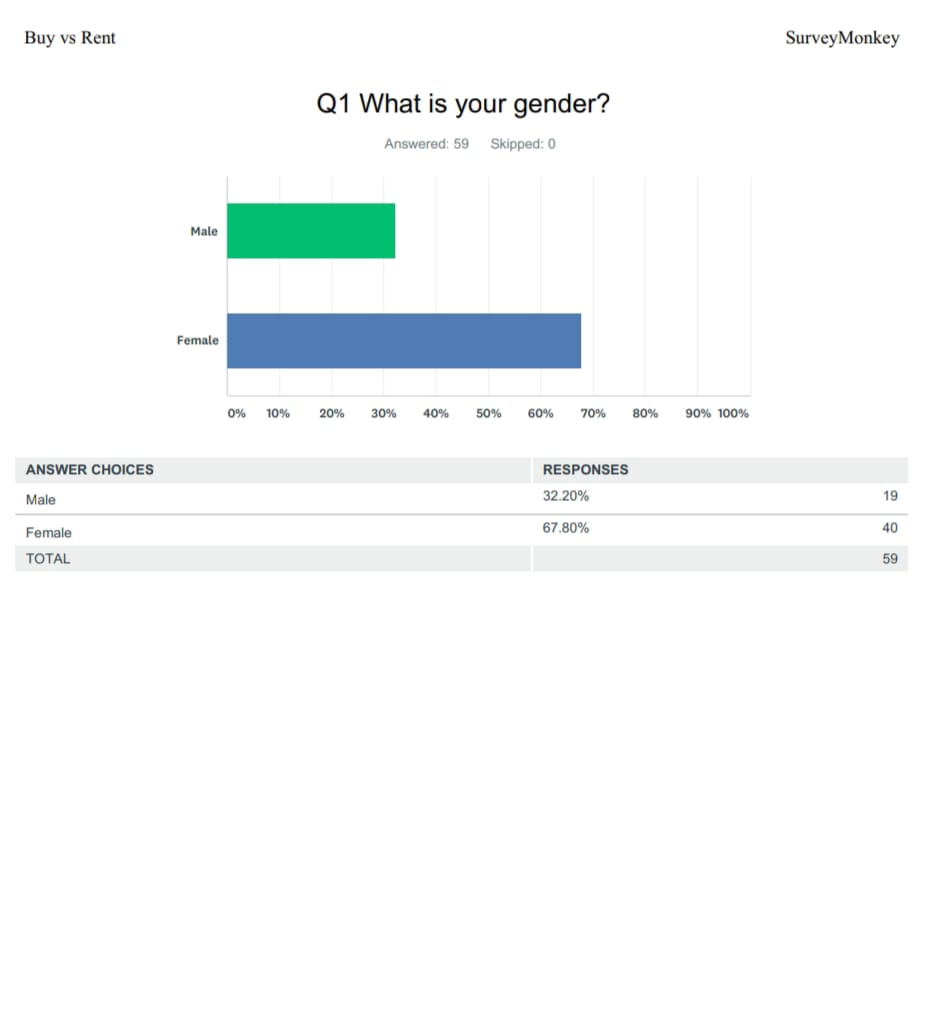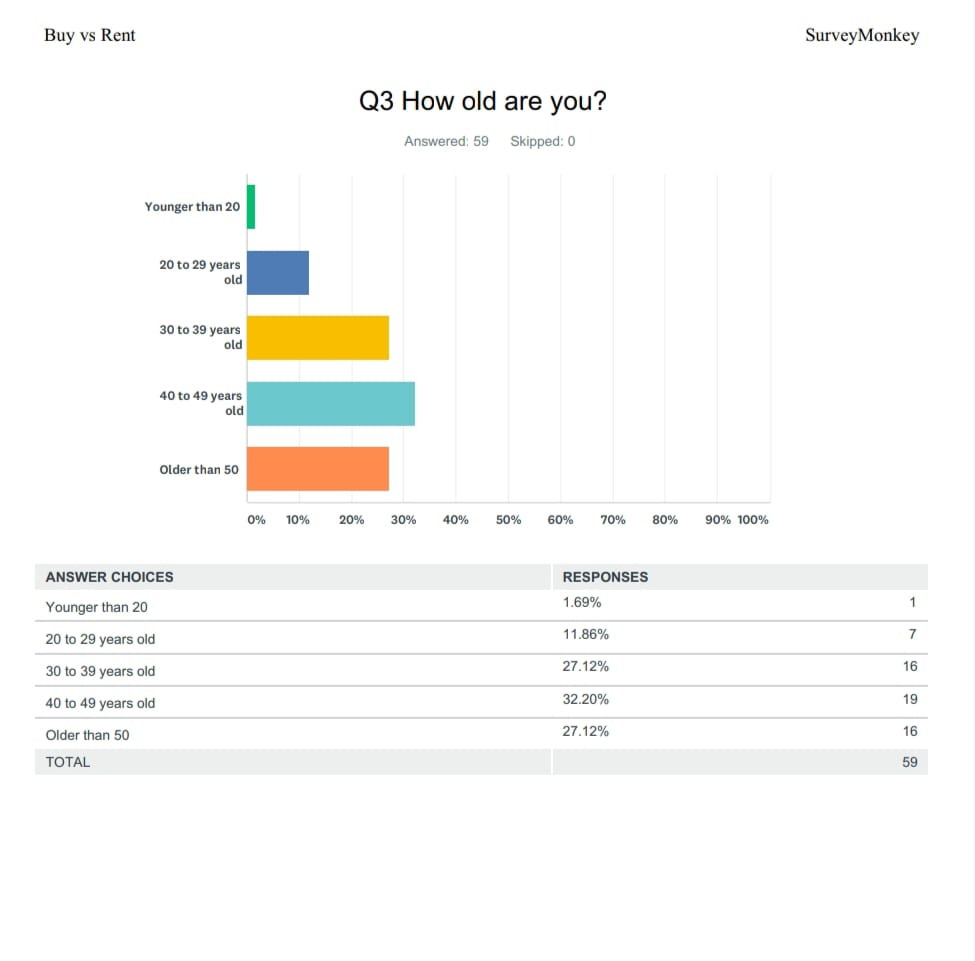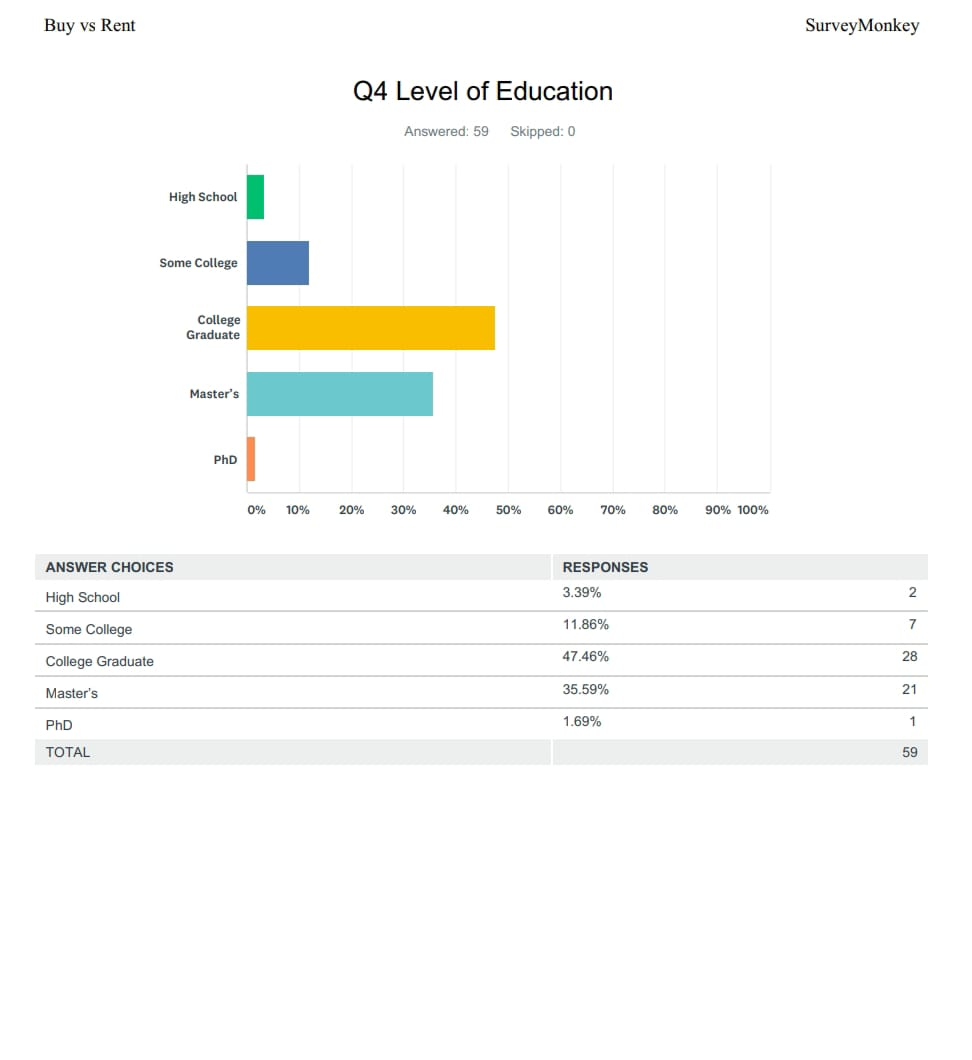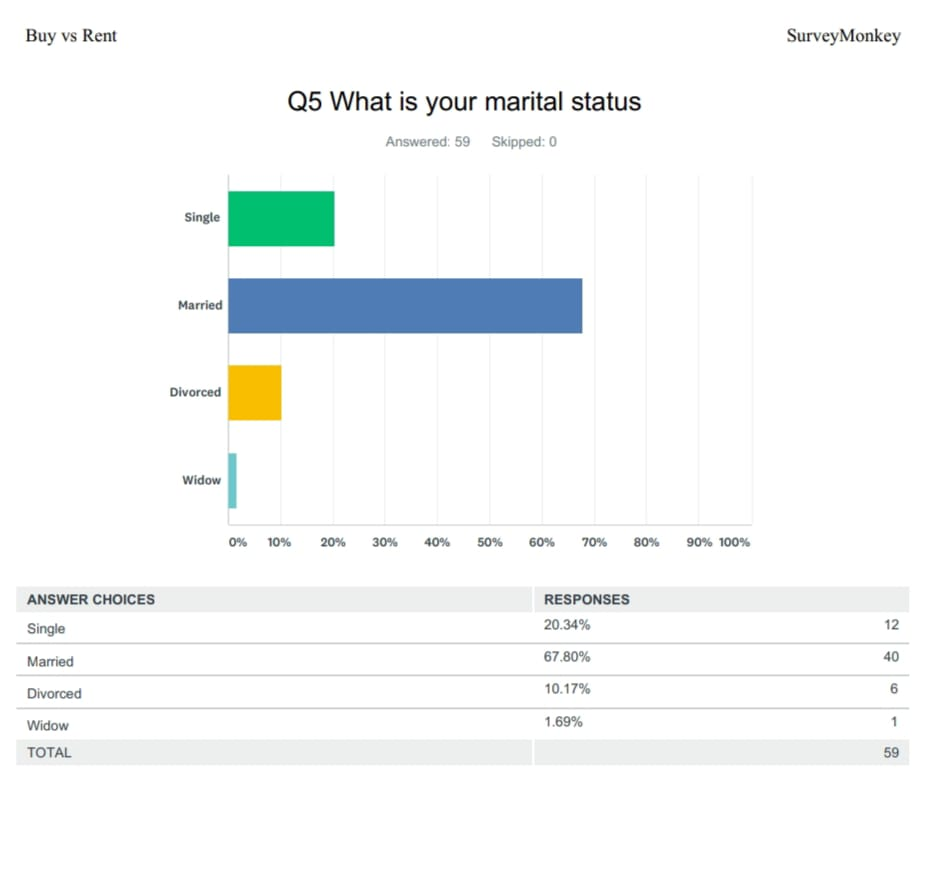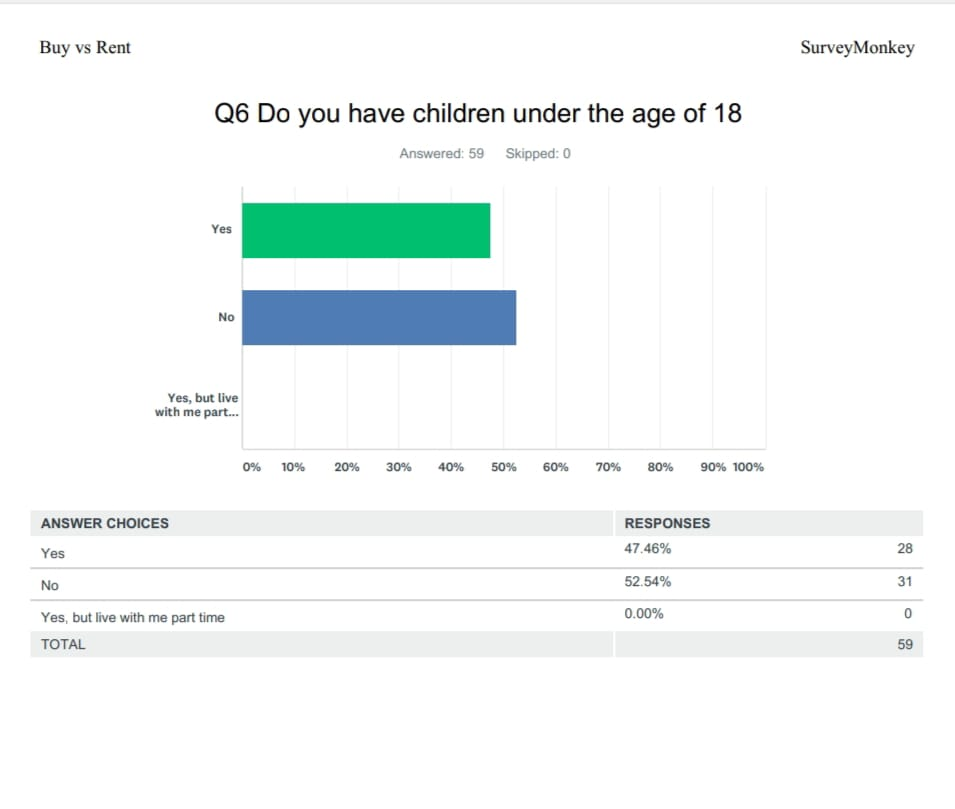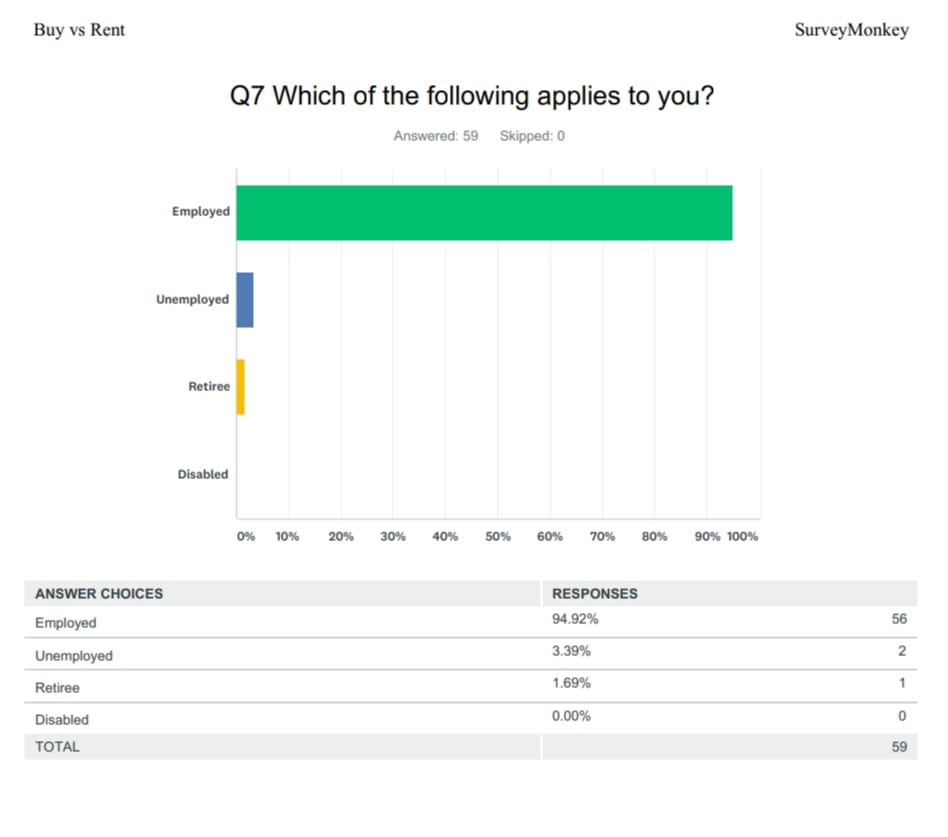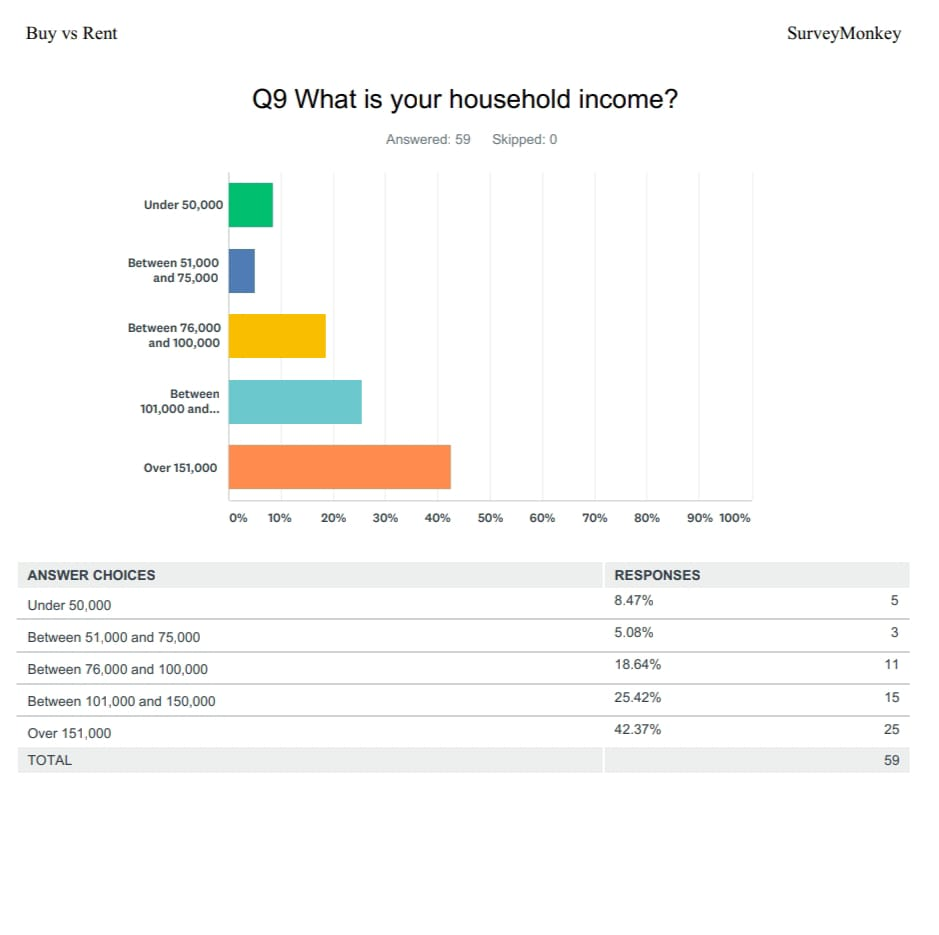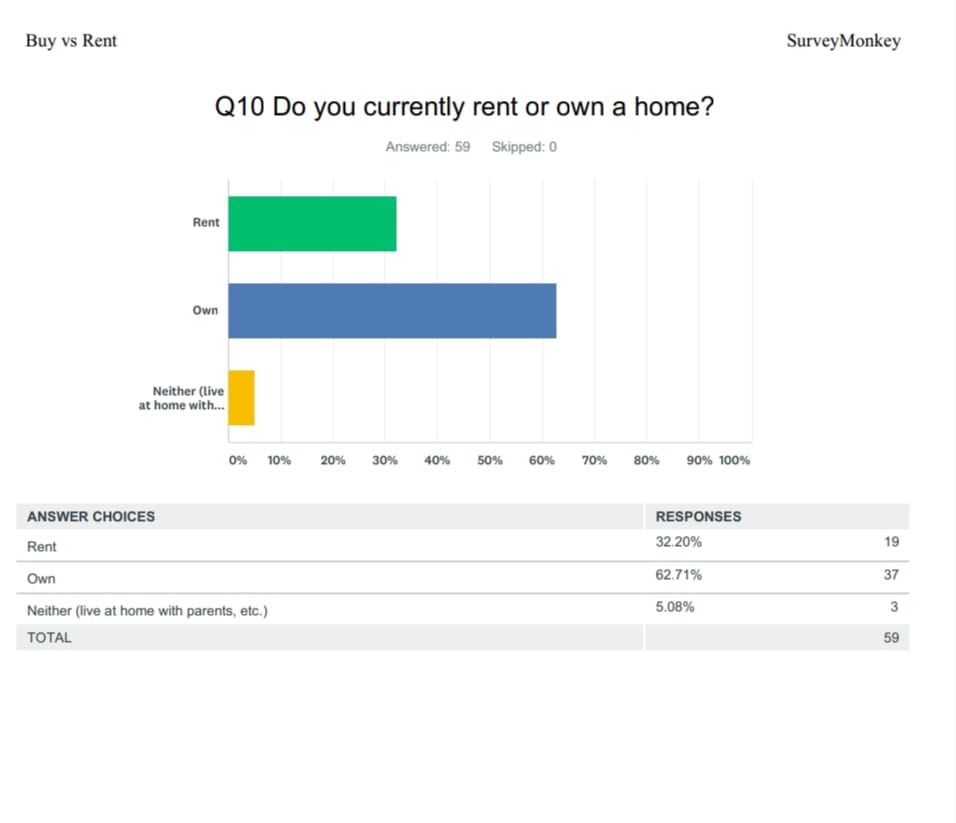1. 99% of z-scores lie between −2.58 and 2.58. The far values are not much different from the mean.
2. 99% of z-scores lie between −2.58 and 2.58. The value differ a lot and quite a few values differ a lot from the mean.
3. There are a lot of differences between the values and the mean.
4. The z-values are quite close and there is not much difference between between the far values and the mean.
5. The z-values are quite close and there is not much difference between between the far values and the mean.
6. 99% of z-scores lie between −2.58 and 2.58. The far values are not much different from the mean.
7. 99% of z-scores lie between −2.58 and 2.58. The far values are not much different from the mean.
8. 99% of z-scores lie between −2.58 and 2.58. The value differ a lot and quite a few values differ a lot from the mean.
9. 99% of z-scores lie between −2.58 and 2.58. The far values are not much different from the mean.
10. The z-values are quite close and there is not much difference between between the far values and the mean.

Looking for Statistics Assignment Help. Whatsapp us at +16469488918 or chat with our chat representative showing on lower right corner or order from here. You can also take help from our Live Assignment helper for any exam or live assignment related assistance.